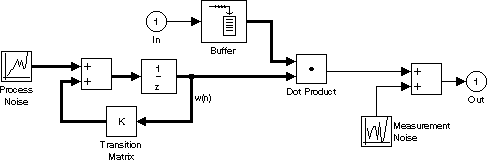Main Content

# Kalman Adaptive Filter (Obsolete)

Compute filter estimates for inputs using Kalman adaptive filter algorithm

## Library

`dspobslib`

•## Description

Note

The Kalman Adaptive Filter block is still supported but is likely to be obsoleted in a future release. We strongly recommend replacing this block with the Kalman Filter block.

The Kalman Adaptive Filter block computes the optimal linear minimum mean-square estimate (MMSE) of the FIR filter coefficients using a one-step predictor algorithm. This Kalman filter algorithm is based on the following physical realization of a dynamic system.The Kalman filter assumes that there are no deterministic changes to the filter taps over time (that is, the transition matrix is identity), and that the only observable output from the system is the filter output with additive noise. The corresponding Kalman filter is expressed in matrix form as

`$\begin{array}{l}g\left(n\right)=\frac{K\left(n-1\right)u\left(n\right)}{{u}^{H}\left(n\right)K\left(n-1\right)u\left(n\right)+{Q}_{M}}\hfill \\ y\left(n\right)={u}^{H}\left(n\right)\stackrel{^}{w}\left(n\right)\hfill \\ e\left(n\right)=d\left(n\right)-y\left(n\right)\hfill \\ \stackrel{^}{w}\left(n+1\right)=\stackrel{^}{w}\left(n\right)+e\left(n\right)g\left(n\right)\hfill \\ K\left(n\right)=K\left(n-1\right)-g\left(n\right){u}^{H}\left(n\right)K\left(n-1\right)+{Q}_{p}\hfill \end{array}$`

The variables are as follows

VariableDescription

n

The current algorithm iteration

u(n)

The buffered input samples at step n

K(n)

The correlation matrix of the state estimation error

g(n)

The vector of Kalman gains at step n

$\stackrel{^}{w}\left(n\right)$

The vector of filter-tap estimates at step n

y(n)

The filtered output at step n

e(n)

The estimation error at step n

d(n)

The desired response at step n

QM

The correlation matrix of the measurement noise

QP

The correlation matrix of the process noise

The correlation matrices, QM and QP, are specified in the parameter dialog by scalar variance terms to be placed along the matrix diagonals, thus ensuring that these matrices are symmetric. The filter algorithm based on this constraint is also known as the random-walk Kalman filter.

The implementation of the algorithm in the block is optimized by exploiting the symmetry of the input covariance matrix K(n) . This decreases the total number of computations by a factor of two.

The block icon has port labels corresponding to the inputs and outputs of the Kalman algorithm. Note that inputs to the In and Err ports must be sample-based scalars with the same complexity. The signal at the Out port is a scalar, while the signal at the Taps port is a sample-based vector.

Block PortsCorresponding Variables
In

u, the scalar input, which is internally buffered into the vector u(n)

Out

y(n), the filtered scalar output

Err

e(n), the scalar estimation error

Taps

$\stackrel{^}{w}\left(n\right)$, the vector of filter-tap estimates

An optional Adapt input port is added when you select the Adapt port check box in the dialog. When this port is enabled, the block continuously adapts the filter coefficients while the Adapt input is nonzero. A zero-valued input to the `Adapt` port causes the block to stop adapting, and to hold the filter coefficients at their current values until the next nonzero Adapt input.

The FIR filter length parameter specifies the length of the filter that the Kalman algorithm estimates. The Measurement noise variance and the Process noise variance parameters specify the correlation matrices of the measurement and process noise, respectively. The Measurement noise variance must be a scalar, while the Process noise variance can be a vector of values to be placed along the diagonal, or a scalar to be repeated for the diagonal elements.

The Initial value of filter taps specifies the initial value $\stackrel{^}{w}\left(0\right)$ as a vector, or as a scalar to be repeated for all vector elements. The Initial error correlation matrix specifies the initial value K(0), and can be a diagonal matrix, a vector of values to be placed along the diagonal, or a scalar to be repeated for the diagonal elements.

## Parameters

FIR filter length

The length of the FIR filter.

Measurement noise variance

The value to appear along the diagonal of the measurement noise correlation matrix. Tunable (Simulink).

Process noise variance

The value to appear along the diagonal of the process noise correlation matrix. Tunable (Simulink).

Initial value of filter taps

The initial FIR filter coefficients.

Initial error correlation matrix

The initial value of the error correlation matrix.

Adapt port

Enables the `Adapt` port.

## References

Haykin, S. Adaptive Filter Theory. 3rd ed. Englewood Cliffs, NJ: Prentice Hall, 1996.

## Supported Data Types

• Double-precision floating point

• Single-precision floating point

## See Also

 LMS Adaptive Filter (Obsolete) DSP System Toolbox RLS Adaptive Filter (Obsolete) DSP System Toolbox

See Noise Cancellation in Simulink Using Normalized LMS Adaptive Filter for related information.

Introduced in R2008b

Watch now